# Conservation of energy

This article is about the law of conservation of energy in physics.

In physics, the law of conservation of energy states that the total amount of energy in an isolated system remains constant. Based on this law, scientists have come to conclude that, in an isolated system, energy is neither created nor destroyed, but it may change form. For example, potential energy can be converted to kinetic energy, and kinetic energy can become thermal energy.

## Contents

By understanding this law, engineers can design machines that can use energy to produce work. For example, thermal energy (or the heat produced by burning a fuel) can be used to drive a turbine, which in turn can be used to generate electricity. In addition, based on this law, one can conclude that perpetual motion machines can operate only if they deliver no energy to their surroundings, and devices that produce more energy than is put into them are impossible. However, with Albert Einstein's formulation of the theory of special relativity, scientists now view the conservation of energy as part of a larger law, namely, the conservation of "mass-energy."

## History

Ancient philosophers had inklings of the conservation of some underlying substance of which everything is made. For example, Thales of Miletus thought it was water.

In 1638, Galileo published his analysis of several situations—including the celebrated "interrupted pendulum"—which can be described (in modern language) as conservatively converting potential energy to kinetic energy and back again. However, Galileo did not state the process in modern terms and again cannot be credited with the crucial insight. It was Gottfried Wilhelm Leibniz during 1676–1689 who first attempted a mathematical formulation of the kind of energy which is connected with motion (kinetic energy). Leibniz noticed that in many mechanical systems (of several masses, mi each with velocity vi),$\sum_{i} m_i v_i^2$

was conserved so long as the masses did not interact. He called this quantity the vis viva or living force of the system. The principle represents an accurate statement of the approximate conservation of kinetic energy in situations where there is no friction. Many physicists at that time held that the conservation of momentum, which holds even in systems with friction, as defined by the momentum: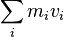$\,\!\sum_{i} m_i v_i$

was the conserved vis viva. It was later shown that, under the proper conditions, both quantities are conserved simultaneously such as in elastic collisions.

It was mainly engineers such as John Smeaton, Peter Ewart, Karl Hotzmann, Gustave-Adolphe Hirn, and Marc Seguin who objected that conservation of momentum alone was not adequate for practical calculation and who made use of Leibniz's principle. The principle was also championed by some chemists such as William Hyde Wollaston. Academics such as John Playfair were quick to point out that kinetic energy is clearly not conserved. This is obvious to a modern analysis based on the second law of thermodynamics but in the eighteenth and nineteenth centuries, the fate of the lost energy was still unknown. Gradually it came to be suspected that the heat inevitably generated by motion under friction, was another form of vis viva. In 1783, Antoine Lavoisier and Pierre-Simon Laplace reviewed the two competing theories of vis viva and caloric theory. Count Rumford's 1798 observations of heat generation during the boring of cannons added more weight to the view that mechanical motion could be converted into heat, and (as importantly) that the conversion was quantitative and could be predicted (allowing for a universal conversion constant between kinetic energy and heat). Vis viva now started to be known as energy, after the term was first used in that sense by Thomas Young in 1807.

The recalibration of vis viva to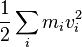$\frac {1} {2}\sum_{i} m_i v_i^2$

which can be understood as finding the exact value for the kinetic energy to work conversion constant, was largely the result of the work of Gaspard-Gustave Coriolis and Jean-Victor Poncelet over the period 1819–1839. The former called the quantity quantité de travail (quantity of work) and the latter, travail mécanique (mechanical work), and both championed its use in engineering calculation.

In a paper, Über die Natur der Wärme, published in the Zeitschrift für Physik in 1837, Karl Friedrich Mohr gave one of the earliest general statements of the doctrine of the conservation of energy in the words: "Besides the 54 known chemical elements there is in the physical world one agent only, and this is called Kraft [energy or work]. It may appear, according to circumstances, as motion, chemical affinity, cohesion, electricity, light and magnetism; and from any one of these forms it can be transformed into any of the others."

A key stage in the development of the modern conservation principle was the demonstration of the mechanical equivalent of heat. The caloric theory maintained that heat could neither be created nor destroyed but conservation of energy entails the contrary principle that heat and mechanical work are interchangeable.

The mechanical equivalence principle was first stated in its modern form by the German surgeon Julius Robert von Mayer. Mayer reached his conclusion on a voyage to the Dutch East Indies, where he found that his patients' blood was a deeper red because they were consuming less oxygen, and therefore less energy, to maintain their body temperature in the hotter climate. He had discovered that heat and mechanical work were both forms of energy, and later, after improving his knowledge of physics, he calculated a quantitative relationship between them.

Meanwhile, in 1843, James Prescott Joule independently discovered the mechanical equivalent in a series of experiments. In the most famous, now called the "Joule apparatus," a descending weight attached to a string caused a paddle immersed in water to rotate. He showed that the gravitational potential energy lost by the weight in descending was equal to the thermal energy (heat) gained by the water by friction with the paddle.

Over the period 1840–1843, similar work was carried out by engineer Ludwig A. Colding though it was little known outside his native Denmark.

Both Joule's and Mayer's work suffered from resistance and neglect but it was Joule's that, perhaps unjustly, eventually drew the wider recognition.

In 1844, William Robert Grove postulated a relationship between mechanics, heat, light, electricity and magnetism by treating them all as manifestations of a single "force" (energy in modern terms). Grove published his theories in his book, The Correlation of Physical Forces. In 1847, drawing on the earlier work of Joule, Sadi Carnot and Émile Clapeyron, Hermann von Helmholtz arrived at conclusions similar to Grove's and published his theories in his book Über die Erhaltung der Kraft (On the Conservation of Force, 1847). The general modern acceptance of the principle stems from this publication.

In 1877, Peter Guthrie Tait claimed that the principle originated with Sir Isaac Newton, based on a creative reading of propositions 40 and 41 of the Philosophiae Naturalis Principia Mathematica. This is now generally regarded as nothing more than an example of Whig history.

## The first law of thermodynamics

Entropy is a function of a quantity of heat which shows the possibility of conversion of that heat into work.

For a thermodynamic system with a fixed number of particles, the first law of thermodynamics may be stated as: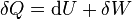$\delta Q = \mathrm{d}U + \delta W\,$, or equivalently,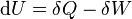$\mathrm{d}U = \delta Q - \delta W\,$,

where$\delta Q$ is the amount of energy added to the system by a heating process,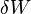$\delta W$ is the amount of energy lost by the system due to work done by the system on its surroundings and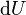$\mathrm{d}U$ is the increase in the internal energy of the system.

The δ's before the heat and work terms are used to indicate that they describe an increment of energy which is to be interpreted somewhat differently than the$\mathrm{d}U$ increment of internal energy. Work and heat are processes which add or subtract energy, while the internal energy$U$ is a particular form of energy associated with the system. Thus the term "heat energy" for$\delta Q$ means "that amount of energy added as the result of heating" rather than referring to a particular form of energy. Likewise, the term "work energy" for$\delta W$ means "that amount of energy lost as the result of work." The most significant result of this distinction is the fact that one can clearly state the amount of internal energy possessed by a thermodynamic system, but one cannot tell how much energy has flowed into or out of the system as a result of its being heated or cooled, nor as the result of work being performed on or by the system. In simple terms, this means that energy cannot be created or destroyed, only converted from one form to another.

For a simple compressible system, the work performed by the system may be written$\delta W = P\,\mathrm{d}V$,

where$P$ is the pressure and$dV$ is a small change in the volume of the system, each of which are system variables. The heat energy may be written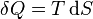$\delta Q = T\,\mathrm{d}S$,

where$T$ is the temperature and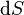$\mathrm{d}S$ is a small change in the entropy of the system. Temperature and entropy are also system variables.

## Mechanics

In mechanics, conservation of energy is usually stated as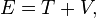$E=T+V,$

where T is kinetic energy and V is potential energy.

Actually, this is the particular case of the more general conservation law: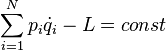$\sum_{i=1}^N p_i \dot{q}_i - L=const$ and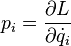$p_i=\frac{\partial L}{\partial \dot{q}_i}$

where L is the Lagrangian function. For this particular form to be valid, the following must be true:

• The system is scleronomous (neither kinetic nor potential energy is an explicit function of time)
• The kinetic energy is a quadratic form with regard to velocities
• The potential energy does not depend on velocities

### Noether's theorem

The conservation of energy is a common feature in many physical theories. From a mathematical point of view it is understood as a consequence of Noether's theorem, which states every symmetry of a physical theory has an associated conserved quantity; if the theory's symmetry is time invariance then the conserved quantity is called "energy." The energy conservation law is a consequence of the shift symmetry of time; energy conservation is implied by the empirical fact that the laws of physics do not change with time itself. Philosophically this can be stated as "nothing depends on time per se." In other words, if the theory is invariant under the continuous symmetry of time translation, then its energy (which is canonical conjugate quantity to time) is conserved. Conversely, theories which are not invariant under shifts in time (for example, systems with time dependent potential energy) do not exhibit conservation of energy—unless one considers them to exchange energy with another, external system so that the theory of the enlarged system becomes time invariant again. Since any time-varying theory can be embedded within a time-invariant meta-theory energy conservation can always be recovered by a suitable re-definition of what energy is. Thus conservation of energy for finite systems is valid in all modern physical theories, such as special and general relativity and quantum theory (including Quantum electrodynamics).

### Relativity

With the formulation of the theory of special relativity by Albert Einstein, energy was proposed to be one component of an energy-momentum 4-vector. Each of the four components (one of energy and three of momentum) of this vector is separately conserved in any given inertial reference frame. Also conserved is the vector length (Minkowski norm), which is the rest mass. The relativistic energy of a single massive particle contains a term related to its rest mass in addition to its kinetic energy of motion. In the limit of zero kinetic energy (or equivalently in the rest frame of the massive particle, or the center-of-momentum frame for objects or systems), the total energy of particle or object (including internal kinetic energy in systems) is related to its rest mass via the famous equation$E=mc^2$. Thus, in special relativity, the rule of conservation of energy was shown to be a special case of a more general rule, alternatively called the conservation of mass and energy, the conservation of mass-energy, the conservation of energy-momentum, the conservation of invariant mass, or now usually just referred to as conservation of energy.

In the theory of general relativity, conservation of energy-momentum is expressed with the aid of a stress-energy-momentum pseudotensor.

### Quantum theory

In quantum mechanics, energy is defined as proportional to the time derivative of the wave function. Lack of commutation of the time derivative operator with the time operator itself mathematically results in an uncertainty principle for time and energy: The longer the period of time, the more precisely energy can be defined (energy and time become a conjugate Fourier pair).

However, there is a deep contradiction between quantum theory's historical estimate of the vacuum energy density in the universe and the vacuum energy predicted by the cosmological constant. The estimated energy density difference is of the order of 10120 times. The consensus is developing that the quantum mechanical derived zero-point field energy density does not conserve the total energy of the universe, and does not comply with our understanding of the expansion of the universe. Intense effort is going on behind the scenes in physics to resolve this dilemma and to bring it into compliance with an expanding universe.

## See also

• Chaos theory
• Thermodynamics
• Uncertainty principle
• Conservation law
• Conservation of mass
• Groundwater energy balance
• Laws of thermodynamics
• Noether's theorem
• Principles of energetics
• Energy transformation
• Energy quality